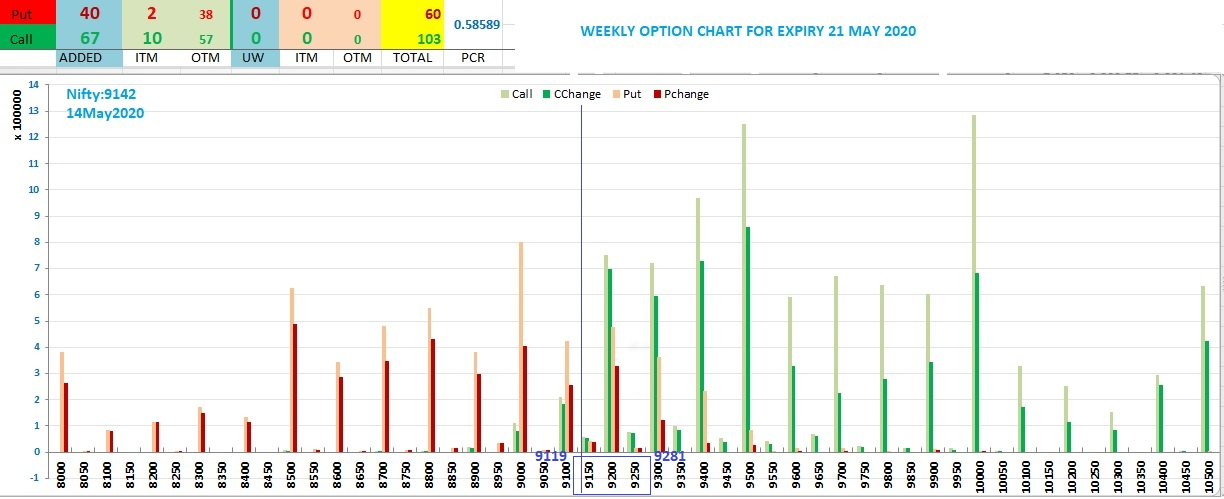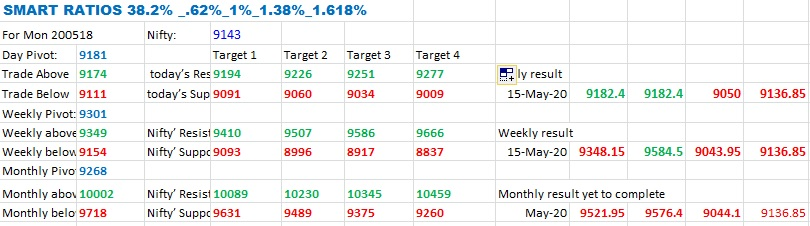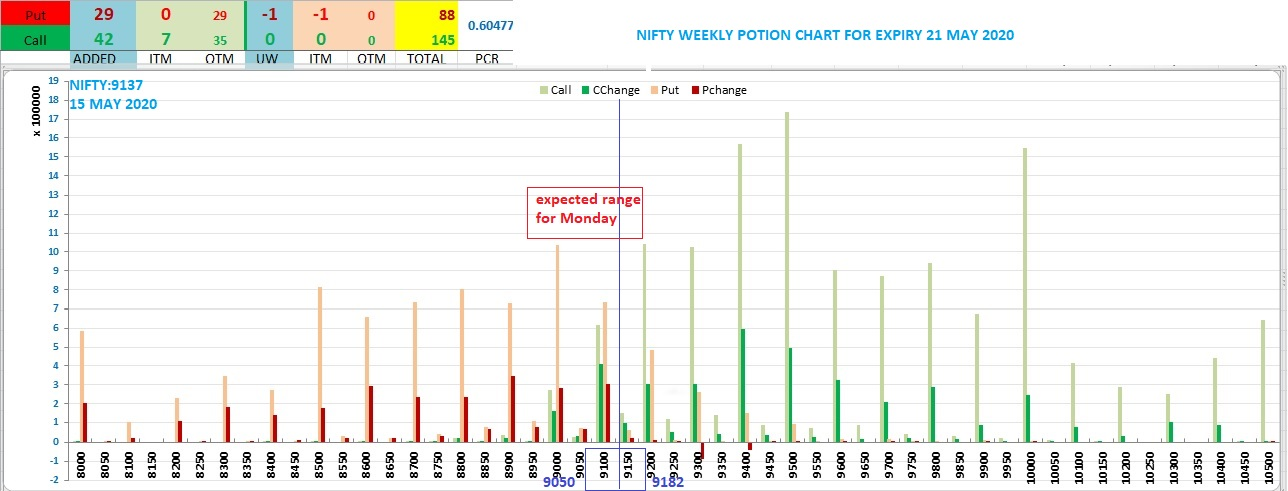# My Picks

##### Well-Known Member
Nifty:9143
open Interest chart for new weekly series: 21 May 2020##### Well-Known Member
Friday, May 15, 2020
How to Use Volatility ratios to find target
Helo Friends,
How to Use Volatility ratios to find target.
Find Volatility by Standard divination of 10 days Closing price
Eg: Nifty take 11 days closing price and prepare a table in excel
1) 11201.75
2) 10989.45 =LN(1/2) =(LN1/2)^2
3) 9955.2 =LN(2/3) ----Do
4) 8745.45 =LN(3/4) ----Do
5) 8660.25 =LN(4/5) ----Do
6) 8083.8 =LN(5/6) ----Do
7) 9111.9 =LN(6/7) ----Do
8) 9266.75 =LN(7/8) ----Do
9) 9154.4 =LN(8/9) ----Do
10) 9859.9 =LN(9/10) ----Do
11) 9251.5 =LN(10/11) =(LN10/11)^2
12) =AVERAGE(LN2 -LN11)=AVERAGE(LN^2 -LN^11)
13) =(avrg og LN - avrg of LN^2) To find variance
14) =SQRT( variance) finally you get the volatility
Once you find the volatility, multiply this with nifty last closing price you will get base value.
Now use the smart ratios .0146 ,0.236, 0.5, 0.618, 1, 1.27, 1.618 etc to find possible targets.
Close price + (base x smart ratio) will be our target.
Be smart to use appropriate ratios based on market conditions.
currently am using the lowest 4 ratios as the market in trading range bound

This is the result we are getting for the day and week ending 15/05/2020##### Well-Known Member
Nifty:9137 what are the possibilities for Monday 18 May 2 020
if trading below Nifty may find sub 9000 level 8950Nifty Weekly option Chart for expiry 21May 2020 as on 15 may##### Well-Known Member
Nifty:9137 what are the possibilities for Monday 18 May 2 020
if trading below Nifty may find sub 9000 level 8950Nifty Weekly option Chart for expiry 21May 2020 as on 15 mayoption Chart##### Well-Known Member
Nifty can find support near 8900 and may bounce back to 9990 level next week

#### timepass

##### Moderator
Nifty can find support near 8900 and may bounce back to 9990 level next week
Is it a typo or are you predicting a 1000 pt upside ?